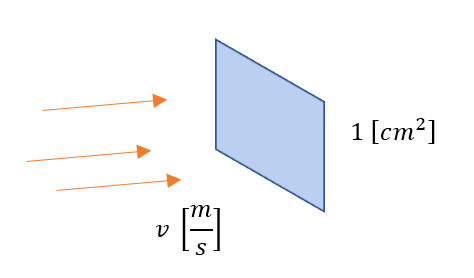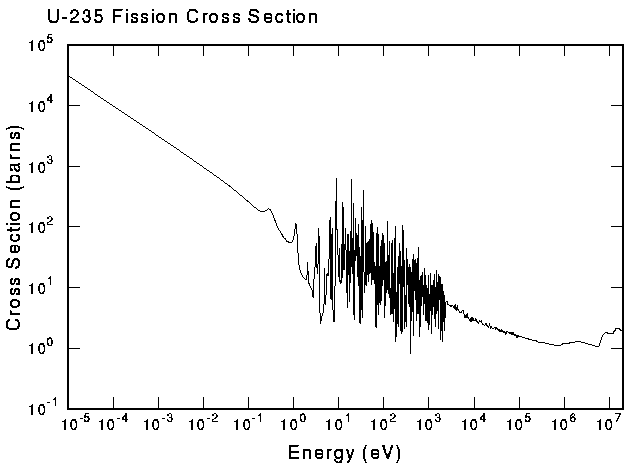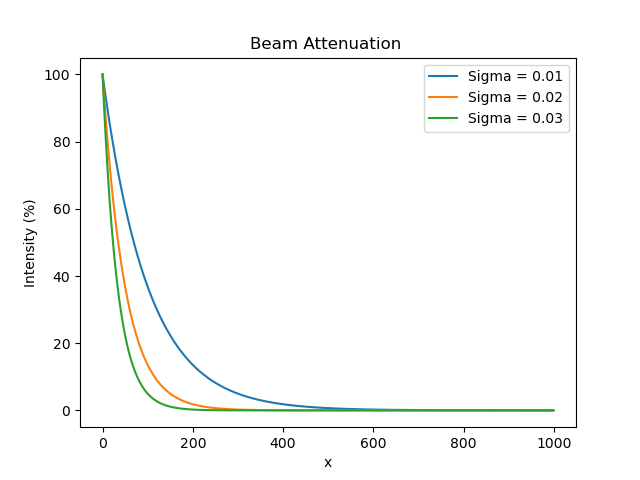# Particle Flux

Researchers use particle beams to study nuclear reactions. For example, bumbarding a fissile material with neturons. Consider a beam of particles with density $$n \; [n/cm^3]$$ and velocity $$v \; [cm/s]$$, travelling in the same direction. The intensity of the beam is:

$$I = nv \; [\cfrac{n}{cm^2 s}]$$

This describes how many particles pass a unit area per second shown in the image below.In a nuclear reactor, there are "beams" of neutrons coming from all directions at different velocities. We can generalize the intensity to the angular flux. The flux at a certain position, $$\vec r$$, from particles of with speed $$v$$ from 3-D direction $$\hat \Omega$$ is:

$$\phi (\vec r, v, \hat \Omega) = n(\vec r, v, \hat \Omega) \cdot v$$

The flux is different at every location due to the geometry of the core and locations of fuel and neutron absorping material. In a CANDU reactor, the flux is naturally peaked in the center but flattened by Adjuster Rods.We can add up all the angular fluxes for a given direction and/or speed:

$$\phi (\vec r, v) = \int_{4\pi} \phi (\vec r, v, \hat \Omega)d\hat \Omega$$

$$\phi (\vec r) = \int \phi (\vec r, v)dv$$

A computer program keeps track of the number of neutrons hitting a small target ($$5 \; [cm^2] * 2 \;[cm]$$). The tallies after $$1 \; [min]$$ are shown below. What are the average fluxes for each energy group and the total average flux at the sample?

 Count Speed $$[\cfrac{m}{s}]$$ $$100,000$$ $$500$$ $$400,000$$ $$1,000$$ $$200,000$$ $$2,000$$

## Cross Sections

Different nuclear reactions occur with a certain probability dependent on the atom, incoming energy of the particles etc. The microscopic cross section represents the probability an incoming particles has to interact with a single atom. It is represented by $$\sigma$$ with units of area $$[cm^2]$$. The macroscopic cross section represents the probability of interacting with any target atom given by $$\Sigma = N\sigma$$ with units of $$[cm^{-1}]$$ where $$N$$ is the atomic density of the target. Somtimes, cross sections are provided in units of $$[barns]$$ where $$1 \; [barn] = 10^{-24} \; [cm^2]$$.

Each nuclear reaction has its own cross section. For example, the scattering, absorption and capture cross sections are identified as $$\Sigma_s, \Sigma_a, \Sigma_{\gamma}$$ respectivley and the total cross section is $$\Sigma_{total} = \sum \Sigma_i$$.

The cross section is dependent on the energy of the incoming particle. In thermal fission, slow neutrons induce fission ins U-235. The fission cross section for uranium tends to be greater when the neutron energy is lower. Hence, moderators are used to thermalize the nuetrons.The microscopic cross section of lead is $$~ 0.2 \; [barn]$$. What is the macroscopic cross section?

## Reaction Rates

The reaction rate describes how many reactions occur per second per unit volume and is found using the formula:

$$RR = \Sigma \phi \; [\cfrac{rxn}{cm^3s}]$$

Since there are different reactions, there are also different reaction rates such as $$RR_s, RR_a, RR_{\gamma}$$.

The reaction rate can be multiplied by the volume to calculate the interaction rate $$IR = RR \cdot V \; [\cfrac{rxn}{s}]$$.

The neutron flux on the periphery of a reactor is $$10^{11} \; [\cfrac{n}{cm^2 s}]$$. A $$12 \; [cm]$$ tall cylindrical target ($$r = 2 \; [cm], \rho = 3.5 \; [\cfrac{g}{cm^3}], MM = 51, \sigma = 9 \; [b])$$. Calculate the interaction rate within the sample.

## Attenuation

A common scenario is to calculate the intensity of a beam over (or at the end) of a target (for example, a shield). We have seen that the reaction rate is proportional to the intensity. As particles undergo nuclear reactions, there are fewer of them left to react. As with radioactive decay, we can write an equation to describe the rate of change of intensity:

$$\cfrac{dI}{dx} = -I \Sigma$$

This differential equation is separable and has the solution:

$$I(x) = I_0 e^{-\Sigma x}$$

The image below shows that larger cross sections cause the intensity to decrease faster.